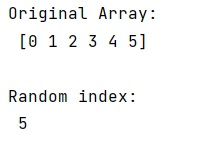# numpy.argmax(): Random tie breaking

Learn about numpy.argmax(), how tie breaking in argmax works?
Submitted by Pranit Sharma, on February 21, 2023

NumPy is an abbreviated form of Numerical Python. It is used for different types of scientific operations in python. Numpy is a vast library in python which is used for almost every kind of scientific or mathematical operation. It is itself an array which is a collection of various methods and functions for processing the arrays.

## numpy.argmax()

The numpy.argmax() is used to return the indices of the maximum values along an axis. It takes an input array, and axis and returns the output as an array of indices into the array.

In this method, tie-breaking between multiple max elements is so that the first element is returned. We need to check if is there a functionality for randomizing tie-breaking so that all maximum numbers have an equal chance of being selected.

For this purpose, we can simply use numpy.random.choice() on flaononzero elements of an array.

Let us understand with the help of an example,

## Python code to demonstrate the numpy.argmax(): random tie breaking

```# Import numpy
import numpy as np

# Creating a array
arr = np.arange(6)

# Display original array
print("Original Array:\n",arr)

# randomizing tie breaking
res = np.random.choice(np.flatnonzero(arr == arr.max()))

# Display result
print("Random index:\n",res)
```

Output: